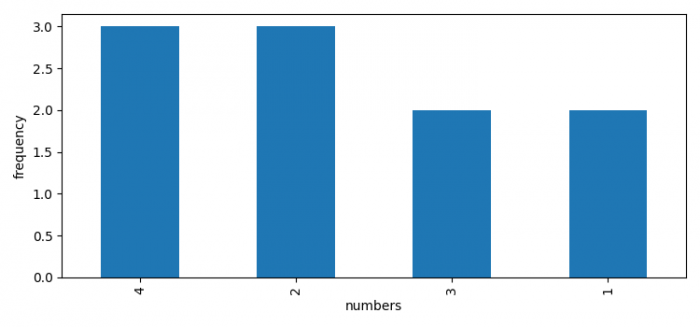# Frequency plot in Python/Pandas DataFrame using Matplotlib

To show a frequency plot in Python/Pandas dataframe using Matplotlib, we can take the following steps −

• Set the figure size and adjust the padding between and around the subplots.
• Create a figure and a set of subplots.
• Make a two-dimensional, size-mutable, potentially heterogeneous tabular data.
• Return a Series containing the counts of unique values.
• To display the figure, use show() method.

## Example

import pandas as pd
from matplotlib import pyplot as plt

plt.rcParams["figure.figsize"] = [7.50, 3.50]
plt.rcParams["figure.autolayout"] = True

fig, ax = plt.subplots()

df = pd.DataFrame({'numbers': [2, 4, 1, 4, 3, 2, 1, 3, 2, 4]})
df['numbers'].value_counts().plot(ax=ax, kind='bar', xlabel='numbers', ylabel='frequency')

plt.show()

## Output